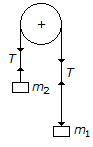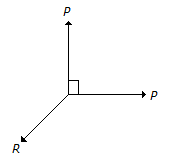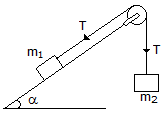Courses

# Test: Engineering Mechanics - 5

## 25 Questions MCQ Test Engineering Mechanics | Test: Engineering Mechanics - 5

Description
This mock test of Test: Engineering Mechanics - 5 for Mechanical Engineering helps you for every Mechanical Engineering entrance exam. This contains 25 Multiple Choice Questions for Mechanical Engineering Test: Engineering Mechanics - 5 (mcq) to study with solutions a complete question bank. The solved questions answers in this Test: Engineering Mechanics - 5 quiz give you a good mix of easy questions and tough questions. Mechanical Engineering students definitely take this Test: Engineering Mechanics - 5 exercise for a better result in the exam. You can find other Test: Engineering Mechanics - 5 extra questions, long questions & short questions for Mechanical Engineering on EduRev as well by searching above.
QUESTION: 1

### According to principle of conservation of energy, the total momentum of a system of masses in any direction remains constant unless acted upon by an external force in that direction.

Solution:

Momentum of system of body is constant when any external force applied on that systems is equation to zero .
because we know ,
change in momentum per unit time is called force.

e.g.
dP/dt=Fext
if Fext =0
then ,
dP/dt =0
it means
Pf-Pi = constant

but external force is constant then
dp/dt =K {Fext =constant =K
dp=Kdt
Pf-pi =Kt
we see
change in momentum is variable.
so, we can say that above statement is false because force is constant hence momentum is variable.

QUESTION: 2

### The friction experienced by a body, when in motion, is known as

Solution:

Friction is categorized in two parts. Dynamics and static friction. Body is moving here so static friction will be eliminated. And not mention about rolling. so rolling friction also eliminated, no concern of force and reactions here so limiting friction is not playing vital role here. Only option remaining is DYNAMIC FRICTION. Or directly we can say wherever body is in motion dynamic friction are there.

QUESTION: 3

### Two balls of equal mass and of perfectly elastic material are lying on the floor. One of the ball with velocity v is made to struck the second ball. Both the balls after impact will move with a velocity

Solution:

Conservation of momentom

mv=m1v1+m2v2

mv=mv`+mv`

v`=v/2

QUESTION: 4

The term 'force' may be defined as an agent which produces or tends to produce, destroys or tends to destroy motion.

Solution:

The answer is correct because motion always equal to multiple of mass and acceleration so we can also said that it produce or tend to produce or destroy tends to destroy motion.

QUESTION: 5

The coefficient of restitution for elastic bodies is one.

Solution:

Coefficient of restitution for elastic bodies is 0 - 1.

Coefficient of restitution for perfect elastic bodies is 1.

QUESTION: 6

The velocity ratio in case of an inclined plane inclined at angle θ to the horizontal and weight being pulled up the inclined plane by vertical effort is

Solution:
QUESTION: 7

The range of projectile on a downward inclined plane is __________ the range on upward inclined plane for the same velocity of projection and angle of projection.

Solution:
QUESTION: 8

A body of weight W is required to move up on rough inclined plane whose angle of inclination with the horizontal is α. The effort applied parallel to the plane is given by(where μ = tanφ = Coefficient of friction between the plane and the body.)

Solution:
QUESTION: 9

The angle of inclination of a vehicle when moving along a circular path __________ upon its mass.

Solution:
QUESTION: 10

If the resultant of two equal forces has the same magnitude as either of the forces, then the angle between the two forces is

Solution:
QUESTION: 11

A smooth cylinder lying on its convex surface remains in __________ equilibrium.

Solution:
QUESTION: 12

Coefficient of friction is the ratio of the limiting friction to the normal reaction between the two bodies.

Solution:
QUESTION: 13

Moment of inertia of a circular section about an axis perpendicular to the section is

Solution:
QUESTION: 14

The time of flight (t) of a projectile on an upward inclined plane is(where u = Velocity of projection, α = Angle of projection, and β = Inclination of the plane with the horizontal.)

Solution:
QUESTION: 15

The unit of angular acceleration is

Solution:
QUESTION: 16

Moment of inertia of a triangular section of base (b) and height (h) about an axis passing through its C.G. and parallel to the base, is

Solution:
QUESTION: 17

If the masses of both the bodies, as shown in the below figure, are reduced to 50 percent, then tension in the string will beSolution:
QUESTION: 18

Which of the following is an equation of linear motion?(where, u and v = Initial and final velocity of the body, a = Acceleration of the body, and s = Displacement of the body in time t seconds.)

Solution:
QUESTION: 19

If a number of forces are acting at a point, theirresultant will be inclined at an angle θ with the horizontal, such that

Solution:
QUESTION: 20The above figure shows the two equal forces at right angles acting at a point. The value of force R acting along their bisector and in opposite direction is

Solution:
QUESTION: 21

The force required to move the body up the plane will be minimum if it makes an angle with the inclined plane __________ the angle of friction.

Solution:
QUESTION: 22

In the shown figure, if the angle of inclination of the plane is increased, then acceleration of the system willSolution:
QUESTION: 23

The total time taken by a projectile to reach maximum height and to return back to the ground, is known as time of flight.

Solution:
QUESTION: 24

The frequency of oscillation of a torsional pendulum is

Solution:
QUESTION: 25

The range of a projectile is maximum, when the angle of projection is

Solution: